# Discrete or continuous modeling ?

Tuesday, we got our conference “Insurance, Actuarial Science, Data & Models” and Dylan Possamaï gave a very interesting concluding talk. In the introduction, he came back briefly on a nice discussion we usually have in economics on the kind of model we should consider. It was about optimal control. In many applications, we start with a one period economy, then a two period economy, and pretend that we can extend it to $n$ period economy. And then, the continuous case can also be considered. A few years ago, I was working on sports game as an optimal effort startegy (within in a game – fixed time). It was with a discrete model, I was running simulations to get an efficient frontier, where coaches might say “ok, now we have enough (positive) difference, and we get closer to the end of the game, so we can ‘lower the effort’ i.e. top players can relax a little bit” (it was on basket-ball games). I asked a good friend of mine, Romuald, to help me on some technical parts of proofs, but he did not like so much my discrete-time model, and wanted to move to continuous time. And for now six years, we keep saying that someday we should get back to that paper….

My initial thoughts were that the difference was really “cultural”: you are either a continuous-time sort of guy, or a discrete-time one (or maybe none of the two, but that’s another problem). He works with stochastic processes, I work with time series. Of course, we can find connections, but most of the time, the techniques are very different. And tuesday, Dylan mentioned a very nice illustration that it’s not necessarily a cultural difference, and sometimes, it is great to move to continuous time. So I wanted to illustrate that idea.

Consider for instance the following curve.

vu = seq(0,1,length=601) vv = sin(vu*pi) plot(vu,vv,type="l",lwd=2)The goal is to find the value of the maximum, numerically. And here, there are two (very) different strategies

• the discrete one: we see a (finite) collection of points – for instance, the graph above is a collection of 601 points (connected with a straight line) – and in that case, we need a standard algorithm (in $O(n)$) to get the value of the maximum
• the continuous one: we see a function $x\mapsto \sin(\pi x)$, and in that case, we use optimization routines

In the second case, use for instance

optim(0,function(x) -sin(pi*x)) $par  0.5$value  -1

For the first case, we can use the standard R function, and see how long it takes to use simulations to get an approximation of the maximum

library(microbenchmark) max_time = function(n) median(microbenchmark(max(sin(runif(n)*pi)))$time) vn = 10^(seq(1,6,length=21)) vt = Vectorize(max_time)(vn) plot(vn,vt/1e9,col="blue",pch=19,type="b",log="xy") but of course, some home-made code can also be used c_max = function(n=100){ x = sin(runif(n)*pi) y = x for(i in 2:length(x)) { if(x[i] &gt; y) { y = x[i] }} return(y)} max_time=function(n) median(microbenchmark(c_max(n))$time) lines(vn,vt/1e9,type="b")We can add that horizontal red line using

abline(h=median(microbenchmark(optim(.5,function(x) sin(pi*x)))$time)/1e9,lty=2,col="red") So, indeed, it looks like computational time to find the maximum in a list of $n$ elements is linear in $n$, i.e. $O(n)$. And R code is faster than home-made code. But also, interestingly, using continus time (based on analysis techniques) can be much faster. So, sometimes, considering continuous time models can be much easier to solve, from a numerical perspective. # Tests, Power and Significance In the mathematical statistics course today, we started talking about tests, and decision rules. To illustrate all the concepts introduced today, we considered the case where we have a sample with . And we want to test against In the course, we’ve seen that we could use a test based on the order statistics . The test would be i.e. if we choose , and if , we choose . From the definition of the first order risk, we can easily get that Thus, the power is then To visualize it, use the following parameters n=5 alpha=.1 theta0=1 Then C1=theta0*(1-alpha)^(1/n) theta=seq(0,2,by=.01) P1=(1-(theta0/theta)^n*(1-alpha))*(theta>C1) plot(theta,P1,type="l",lwd=2,col="blue",xlab="",ylab="Power")Note that, so far, we did never consider the maximum of our sample. Assume that the maximum is , then we can compute the -value, Here it is PV=(1-theta^n)*(theta<=1) plot(theta,PV,type="l",lwd=2,col="blue",xlab="",ylab="p-value")Now, why not consider another test, based on the minimum (since we have the distribution of the minimum of a sample from a uniform distribution). The test is the same as before but here, the threshold is The power of the test is here This test has the same significance level (by construction), but the power of the test is clearly lower than the one we got using the maximum of our sample, when C2=theta0*(1-alpha^(1/n)) P2=(1-(theta0/theta)*(1-alpha^(1/n)))^n*(theta>C2) lines(theta,P2,type="l",lwd=2,col="red")Why not consider a test based on ? The problem is that we need the distribution (more specifically the survival distribution) of . We can compute it, numerically. But that might be painful. An alternative is to consider some approximation, based on the central limit theorem, i.e. Our test is based on , and to get the same significance as before, use The power of the test is then Here it is mu=2*(theta0/2) s2=2^2*(theta0^2/12)/n C3=qnorm(1-alpha,mu,sqrt(s2)) (P=1-pnorm(C3,theta,sqrt(s2)))*(theta>C3) lines(theta,P)Observe here that the test based on the maximum is not more powerful than the one based on the average (I just wonder if it could be due to the Gaussian approximation…). # How old is the oldest person you know? Last week, we had a discussion with some colleagues about the fact that – in order to prepare for the SOA exams – we did not have time (so far) to mention results on extreme values in our actuarial program. I did gave an introduction in my nonlife actuarial models class, but it was only an introduction, in three hours, in order to illustrate reinsurance pricing. And I told my students that if they wanted to know more about extreme values, they should start a master program in actuarial science and finance, since I will give a course on extremes (and copulas) next winter. But actually, extreme values are everywhere ! For instance, there is a Prudential TV commercial where has people place large, round stickers on a number line to represent the age of the oldest person they know. This forms some kind of histogram. The message is to have Prudential prepare you to have adequate money for all these years. And actually, anyone can add his or her own sticker at the Prudential website.Patrick Honner, on his blog (http://mrhonner.com/…), did mention this interesting representation. But this idea is not new, as mentioned in a post, published three years ago. In 1932, Emil Gumbel gave a talk in France on the “âge limite“. And as he wrote it “on peut donc supposer que la distribution de l’âge limite – c’est à dire la probabilité que cet âge ait une valeur donnée – soit Gaussienne“. In 1932 (not aware of Fisher and Tippett work, he thought that the limiting distribution for a maximum would be Gaussian). But a few years after, he read about Fisher’s work, and observed also that “la distribution d’une valeur extrêmes peut être représentée pour un nombre suffisant d’observations par la formule doublement exponentielle, pourvu que la distribution initiale se comporte asymptotiquement comme une exponentielle. La formule devient rigoureuse si la distribution initiale est exponentielle“, as he wrote in 1935. And in 1937, he wrote a paper on “les centennaires” that can also be related to the work of Bortkiewicz on rare events. One should also mention one of the most important paper in extreme value theory, published in 1974 by Balkema and de Haan, on Residual Life Time at Great Age. Because in this experiment, the question is “How Old is the Oldest Person You Know?“, so it is the distribution of a maximum. And from Fisher-Tippett theorem, if we assume that the age is bounded (and that there exists some finite upper limit), then the limiting distribution for the maxima (or to be more rigorous, a affine transformation of the maxima) should be Weibull distribution. And this is what it looks like > plot(-x,dweibull(x,2.25,4),type="l",lwd=2)As an actuary, the only thing I know about demography, is the distribution of the age of death. For instance, consider the following French life table > alive <- read.table( + "https://perso.univ-rennes1.fr/arthur.charpentier/TV8890.csv", + sep=";",header=TRUE)$Lx
> nb= -diff(alive)
> ages=0:110
> plot(ages,nb,type="h")This is the distribution of the age of the death in a given population. Which is not the same as the distribution mentioned above! What we look for is the following: given that someone is alive, what could be the distribution of his-her age ? Actually, if we assume that the yearly number of birth is constant with time (as well as death probability), then we can compute easily to number of people of age  : we take everyone born (exactly) years ago, and remove all those who died at at , , etc. So the function should be

> probadeath=nb/sum(nb)
> surv=Vectorize(nbx)(ages)
> distrage=surv/sum(surv)

which looks likeBut this assumption of constant number of birth is not that relevent. And actually, what we need is the distribution of the age within a population… This is a population pyramid, actually. The French one can be downloaded from http://www.insee.fr/fr/ppp/bases-de-donnees/….

> population <- read.table("popinsee2007.csv",sep=";",header=TRUE)$POPTOT07 > ages=0:107 > plot(ages,population/sum(population),type="h")(the red line being the one obtained previously, using some natality assumptions). Now, let us use this population to generate acquaintances. > agemax=function(nsim=1000,size=20){ + agemax=rep(NA,nsim) + for(i in 1:nsim){ + X=sample(ages,prob=population/sum(population),size=size,replace=TRUE) + agemax[i]=max(X)} + return(agemax)} Here, we assume that everyone knows 20 other people, randomly chosen in the entire population, then we return the age of the oldest. And we do that for 1,000 people. Here is the distribution, we obtain > XS=agemax(10000,20) > plot(table(XS)/length(XS),type="h",xlim=c(0,108))where the red line is a Weibull distribution (a transformed one, actually, since in extremely value theory, the distance to the upper bound of the distribution has a Weibull density), > library(MASS) > fit=fitdistr(108-XS,dweibull,list(shape=1,scale=1)) > lines(ages,dweibull(108-ages,fit$estimate,fit\$estimate),col="red")

Which is quite close to the distribution obtained in the commercial, don’t you think ? But still, it should be possible to be more accurate, since people should think of their parents, or grandparents. So I guess it could be possible to build a more accurate algorithm, to get something closer to the distribution obtained on the Prudential website. But first, let us wait to have more stickers, more observations… and then I’ll be back to play with it !

# Fisher-Tippett theorem and limiting distribution for the maximum

Tomorrow, we will discuss Fisher-Tippett theorem. The idea is that there are only three possible limiting distributions for normalized versions of the maxima of i.i.d. samples. For bounded distribution, consider e.g. the uniform distribution on the unit interval, i.e.on the unit interval. Letand. Then, for alland,i.e. the limiting distribution of the maximum is Weibull’s.

set.seed(1)
s=1000000
n=100
M=matrix(runif(s),n,s/n)
V=apply(M,2,max)
bn=1
an=1/n
U=(V-bn)/an
hist(U,probability=TRUE,,col="light green",
xlim=c(-7,1),main="",breaks=seq(-20,10,by=.25))
u=seq(-10,0,by=.1)
v=exp(u)
lines(u,v,lwd=3,col="red")For heavy tailed distribution, or Pareto-type tails, consider Pareto samples, with distribution function. Letand, thenwhich means that the limiting distribution is Fréchet’s.

set.seed(1)
s=1000000
n=100
M=matrix((runif(s))^(-1/2),n,s/n)
V=apply(M,2,max)
bn=0
an=n^(1/2)
U=(V-bn)/an
hist(U,probability=TRUE,col="light green",
xlim=c(0,7),main="",breaks=seq(0,max(U)+1,by=.25))
u=seq(0,10,by=.1)
v=dfrechet(u,shape=2)
lines(u,v,lwd=3,col="red")For light tailed distribution, or exponential tails, consider e.g. a sample of exponentially distribution variates, with common distribution function. Letand, theni.e. the limiting distribution for the maximum is Gumbel’s distribution.

library(evd)
set.seed(1)
s=1000000
n=100
M=matrix(rexp(s,1),n,s/n)
V=apply(M,2,max)
(bn=qexp(1-1/n))
log(n)
an=1
U=(V-bn)/an
hist(U,probability=TRUE,col="light green",
xlim=c(-2,7),ylim=c(0,.39),main="",breaks=seq(-5,15,by=.25))
u=seq(-5,15,by=.1)
v=dgumbel(u)
lines(u,v,lwd=3,col="red")Consider now a Gaussiansample. We can use the following approximation of the cumulative distribution function (based on l’Hopital’s rule)as. Letand. Then we can getas. I.e. the limiting distribution of the maximum of a Gaussian sample is Gumbel’s. But what we do not see here is that for a Gaussian sample, the convergence is extremely slow, i.e., with 100 observations, we are still far away from Gumbel distribution,and it is only slightly better with 1,000 observations,

set.seed(1)
s=10000000
n=1000
M=matrix(rnorm(s,0,1),n,s/n)
V=apply(M,2,max)
(bn=qnorm(1-1/n,0,1))
an=1/bn
U=(V-bn)/an
hist(U,probability=TRUE,col="light green",
xlim=c(-2,7),ylim=c(0,.39),main="",breaks=seq(-5,15,by=.25))
u=seq(-5,15,by=.1)
v=dgumbel(u)
lines(u,v,lwd=3,col="red")Even worst, consider lognormal observations. In that case, recall that if we consider (increasing) transformation of variates, we are in the same domain of attraction. Hence, since, iftheni.e. using Taylor’s approximation on the right term,This gives us normalizing coefficients we should use here.

set.seed(1)
s=10000000
n=1000
M=matrix(rlnorm(s,0,1),n,s/n)
V=apply(M,2,max)
bn=exp(qnorm(1-1/n,0,1))
an=exp(qnorm(1-1/n,0,1))/(qnorm(1-1/n,0,1))
U=(V-bn)/an
hist(U,probability=TRUE,col="light green",
xlim=c(-2,7),ylim=c(0,.39),main="",breaks=seq(-5,40,by=.25))
u=seq(-5,15,by=.1)
v=dgumbel(u)
lines(u,v,lwd=3,col="red")# “standardized” version of the maximum

For the first homework, there was a tricky question in problem 29, chapter 5. Here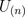is the maximum of n random variables i.i.d. uniformly distributed on the unit interval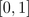. I gave a hint last week about the cumulative distribution function for the maximum, i.e.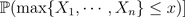is equal to the probability that all are smaller than,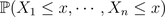Then, we use independent to obtain that this probability is a product, of equal quantities since all random variables are identically distributed, i.e.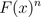Then, the exercise ask the followingi.e. find a standardized version of that the maximum so that the cumulated distribution of that standardized version has a (non degenerated) limiting value. A hint is given in the answers, at the end of the book,Actually, the question is not that simple (see here for the history of that question).
What I said during the course is that if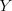is a random variable with finite variance, thenis a standardized (or normalized) version of, in the sense that it is centered, i.e.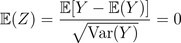and with a unit variance, i.e.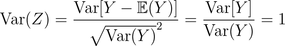This is the kind of standardization (or normalization) that is used in the central limit theorem i.e. it is interesting when we study the core of our distribution (i.e. the mean).
Here we focus on the maxima (not on the expected value). Note that here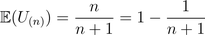while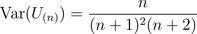(up to some typing mistakes). Thus, our previous standardization would be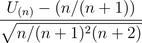that can be simplified as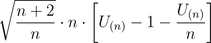Hence, that random variable can be approximated by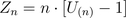since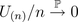as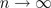. Here, it is then possible to get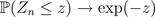since if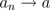, then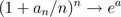(see the prof of the central limit theorem we got a few days ago).
But this is usually not the way we work with maxima. Actually, Fréchet, Fisher, Tippett, Gnedenko proved that the appropriate standardization to work with maxima is to consider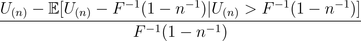where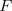is the cumulative distribution of the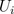‘s (the random variables used to build up the maximum). This work since the‘s have a finite support, i.e. theare bounded, with an upper limit (here 1).
Note that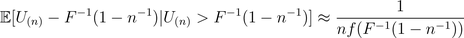assuming that the density associated withexists. Hence, here the standardization becomes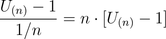which is exactly the one that John Rice is suggesting… And the proper motivation comes from extreme value theory, but it is a bit far away from what we shall see in that course…